# The terminals of a battery are connected across two different resistors in series. Which of the...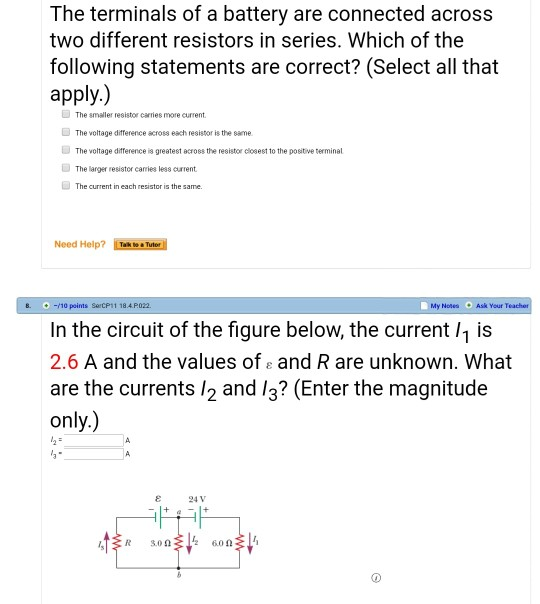The terminals of a battery are connected across two different resistors in series. Which of the following statements are correct? (Select all that apply.) The smaller resistor carries more current The voltage difference across each resistor is the same The voltage difference is greatest across the resistor closest to the positive terminal The larger resistor carries less current The current in each resistor is the same. Need Help? ǐwawaniel kor Techer B. -110 points SerCP11 18.4 P022 In the circuit of the figure below, the current /1 is 2.6 A and the values of e and R are unknown. What are the currents /2 and 13? (Enter the magnitude only.) 13- 24V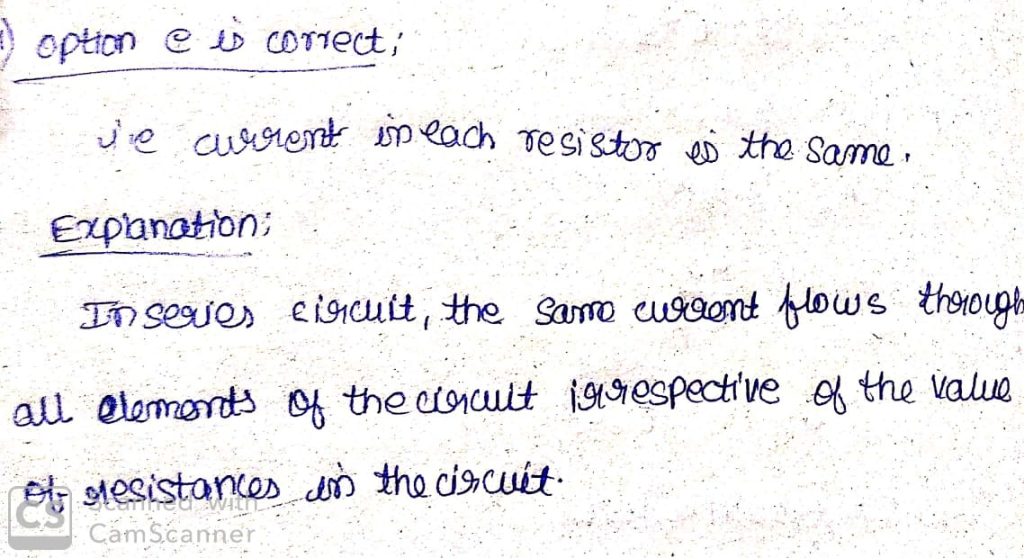#### Earn Coin

Coins can be redeemed for fabulous gifts.

Similar Homework Help Questions
• ### When two resistors are connected in parallel across a battery of unknown voltage, one resistor...

When two resistors are connected in parallel across a battery of unknown voltage, one resistor carries a current of 5.8 A while the second carries a current of 2.9 A.What current will be supplied bu the same battery if these two resistors are connected to it in series?

• ### A battery is connected across a series combination of two identical resistors. If the potential difference...

A battery is connected across a series combination of two identical resistors. If the potential difference across the terminals is V and the current in the battery is i, then: Which of these statement are true? The potential difference across each resistor is V and the current in each resistor is i The potential difference across each resistor is V/2 and the current in each resistor is i The potential difference across each resistor is V and the current in...

• ### 1. A battery is connected to a light bulb, lighting the bulb. Where in the circuit...

1. A battery is connected to a light bulb, lighting the bulb. Where in the circuit is the current the greatest? A) inside the battery B) it's the same at all these points C) inside the light bulb filament D) between the light bulb and the negative terminal of the battery E) between the positive terminal of the battery and the light bulb 2. The emf of a battery is: A) its internal resistance B) the voltage difference it provides...

• ### When two or more resistors are connected in parallel to a battery, the voltage across each...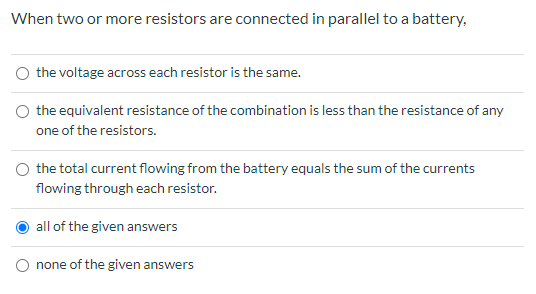When two or more resistors are connected in parallel to a battery, the voltage across each resistor is the same. the equivalent resistance of the combination is less than the resistance of any one of the resistors. the total current flowing from the battery equals the sum of the currents flowing through each resistor. all of the given answers O none of the given answers

• ### A circuit consists of two resistors connected in series with a power supply, as shown in...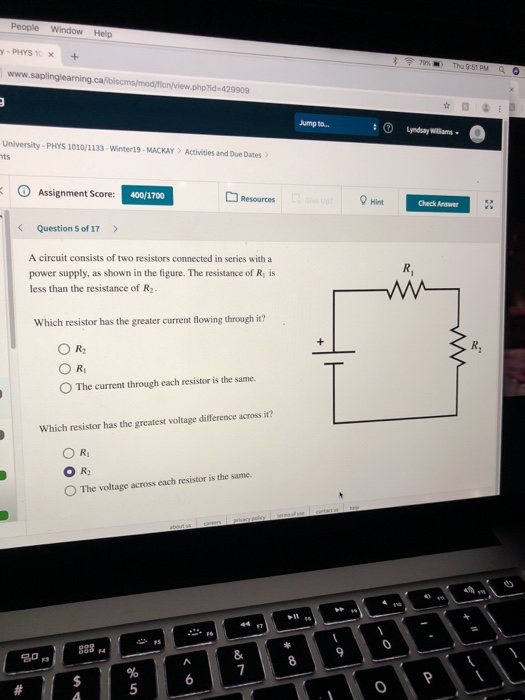A circuit consists of two resistors connected in series with a power supply, as shown in the figure. The resistance of ?1 is less than the resistance of ?2. A series circuit with two resistors R subscript 1 and R subscript 2. R subscript 1 is connected to the positive terminal of the power supply, and is the first resistor in the circuit. Which resistor has the greater current flowing through it? ?2 ?1 The current through each resistor is...

• ### Two resistors A and B are connected in series to a 6.0-V battery; the voltage across...

Two resistors A and B are connected in series to a 6.0-V battery; the voltage across resistor A is 2.0 V. When A and B are connected in parallel across a 6.0-V battery, the current through B is 2.0 A. What is the resistance of resistor A?

• ### Part A Consider three resistors with unequal resistances connected in series to a battery. Which of...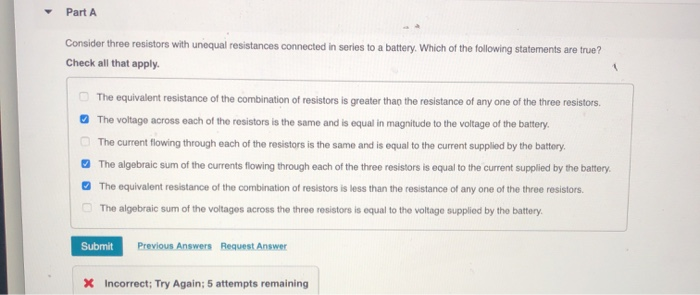Part A Consider three resistors with unequal resistances connected in series to a battery. Which of the following statements are true? Check all that apply. The equivalent resistance of the combination of resistors is greater than the resistance of any one of the three resistors The voltage across each of the resistors is the same and is equal in magnitude to the voltage of the battery. The current flowing through each of the resistors is the same and is equal...

• ### Two resistors are connected in parallel across an ideal 12.0 V battery. Resistor A has a...

Two resistors are connected in parallel across an ideal 12.0 V battery. Resistor A has a value of 28 Ω and resistor B carries a current of 0.2505 A. What is the total power dissipated in the circuit?Two resistors are connected in parallel across an ideal 12.0 V battery. Resistor A has a value of 28 Ω and resistor B carries a current of 0.2505 A. What is the total power dissipated in the circuit?

• ### A circuit consists of two resistors connected in series with a power supply, as shown in...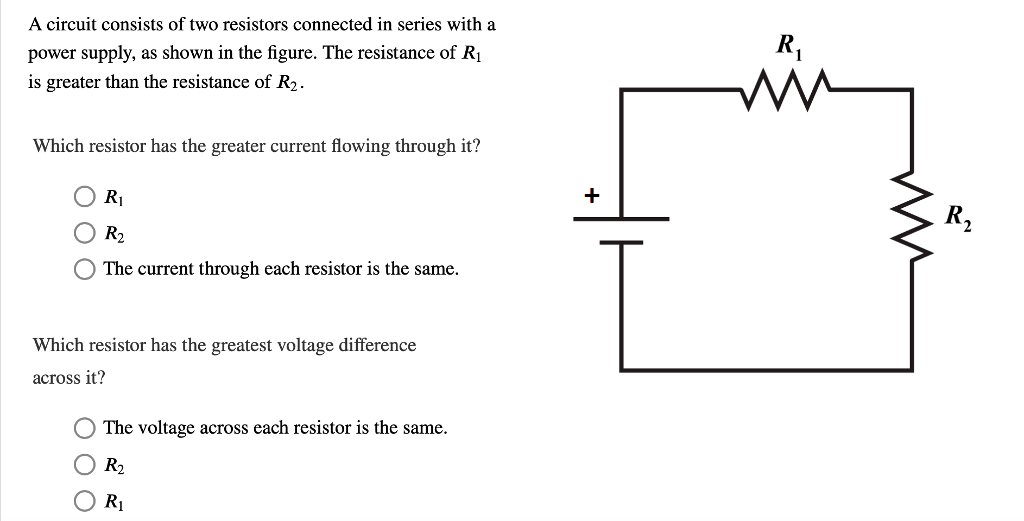A circuit consists of two resistors connected in series with a power supply, as shown in the figure. The resistance of Ri is greater than the resistance of R2 Which resistor has the greater current flowing through it? O The current through each resistor is the same. Which resistor has the greatest voltage difference across it? The voltage across each resistor is the same.

• ### When you connect an unknown resistor across the terminals of a 1.50V AAA battery having negligible...

When you connect an unknown resistor across the terminals of a 1.50V AAA battery having negligible internal resistance, you measure a current of 18.3mA flowing through it. What is the resistance of this resistor? If you now place the resistor across the terminals of a 12.9V car battery having no internal resistance, how much current will flow? You now put the resistor across the terminals of an unknown battery of negligible internal resistance and measure a current of 0.459A flowing...